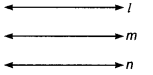# Prove that the two lines which are both parallel to the same line

Prove that the two lines which are both parallel to the same line, are parallel to each other.

Given Three lines l, m and n in a plane such that
l ll n and m ll n.
To prove l ll m
Proof If possible, let l is not parallel to m. Then, l and m should intersect at a unique point, say P.
Thus, through a point P, outside n, there are two lines l and m, both parallel to n.This contradicts the parallel lines axiom.
So, our assumption was wrong.
Hence, l ll m